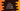# C++ program to find the first and the last digit of a number## Introduction :

In this C++ tutorial, we will learn how to find the first and the last digits of a user-given number. For example, if the number is 12459, it will print 9 as the last digit and 1 as the first digit.

Our program will take the number as an input from the user and print out the result.

### C++ program to find the first and the last number:

Below is the complete C++ program :

``````#include <iostream>
using namespace std;

int main()
{
int number;
cout << "Enter a number : " << endl;
cin >> number;

cout << "Last digit is : " << number % 10 << endl;

while(number >= 10){
number = number/10;
}

cout<< "First digit is : "<< number << endl;
return 0;
}``````

### Explanation :

Here,

• we have one integer variable number to hold the user input number. Using cout and cin, we are reading one value from the user. The program will ask the user to enter a number, it will read it and store it in the variable number.
• The last cout prints the last digit of the number, i.e. number % 10. The modulo operation x % y returns the remainder if x is divided by y. If we do x % 10 for a number x, it will return the last digit of that number. That’s it.
• To find the first digit, we are removing all digits of the number one by one from right to left. We are dividing the number by 10 in the while loop. That will remove the rightmost digit on each step. We are doing it until the current value becomes less than 10. That is the first digit.

Following are some sample outputs of the above program :

``````Enter a number :
129897
Last digit is : 7
First digit is : 1

Enter a number :
98976790
Last digit is : 0
First digit is : 9

Enter a number :
99999999
Last digit is : 9
First digit is : 9``````